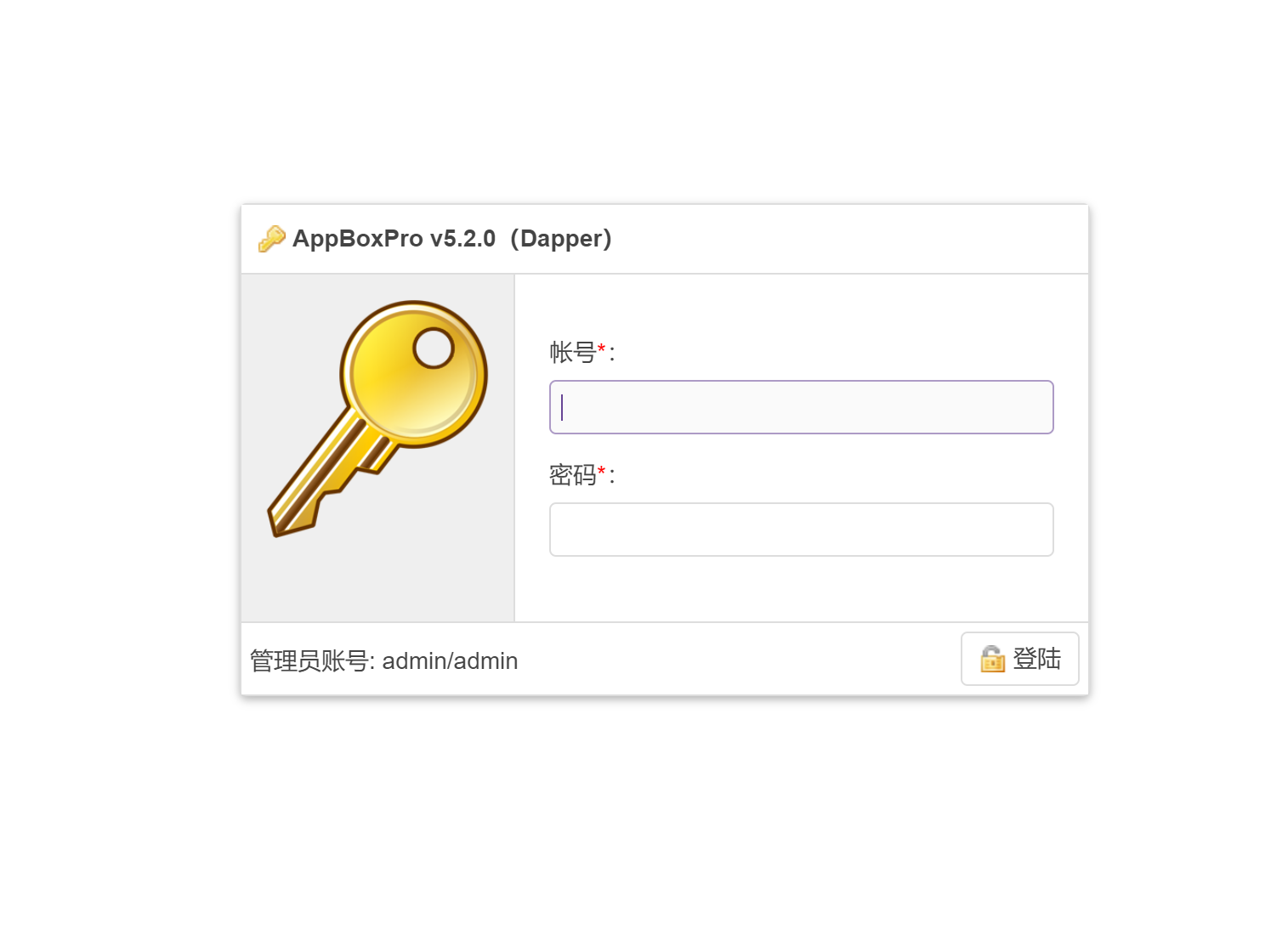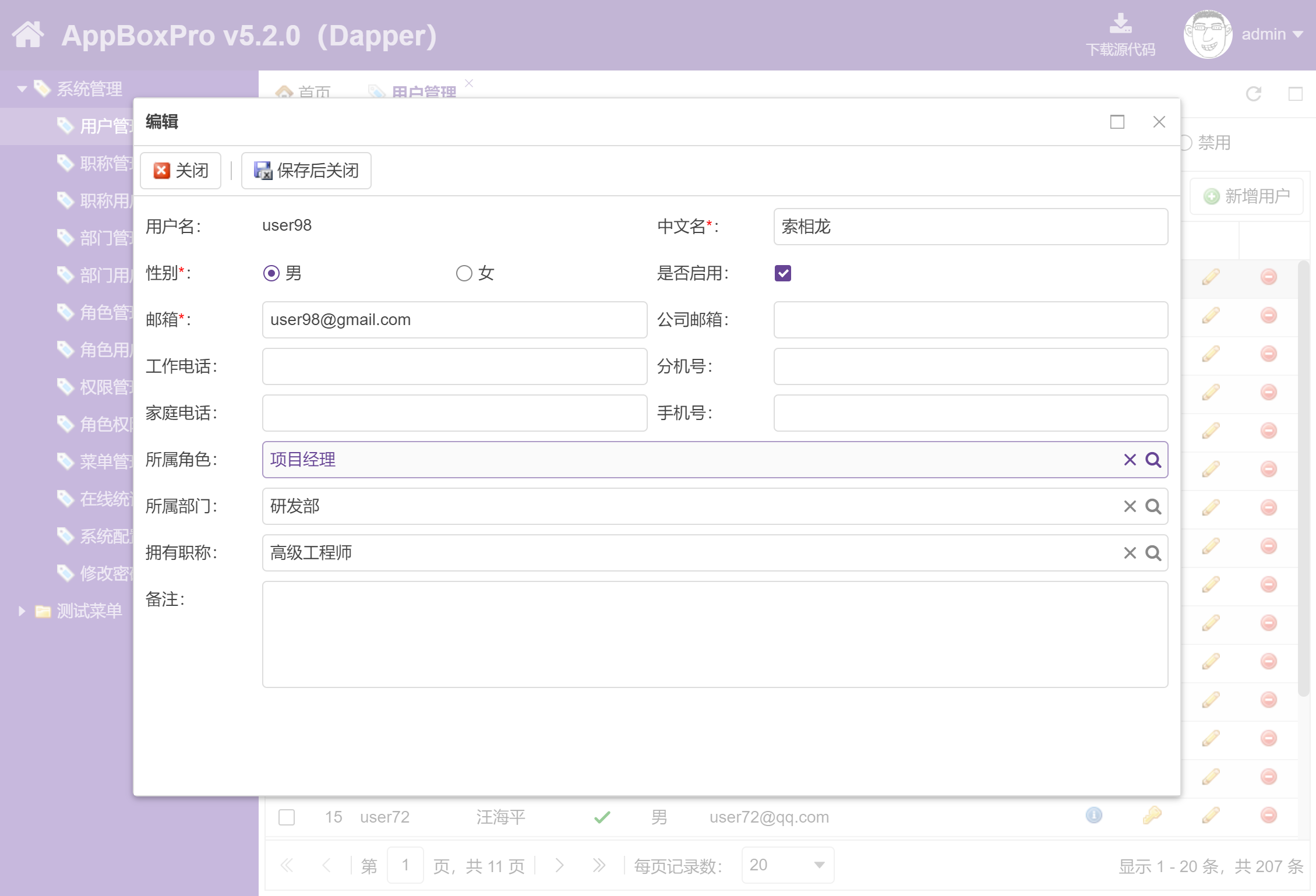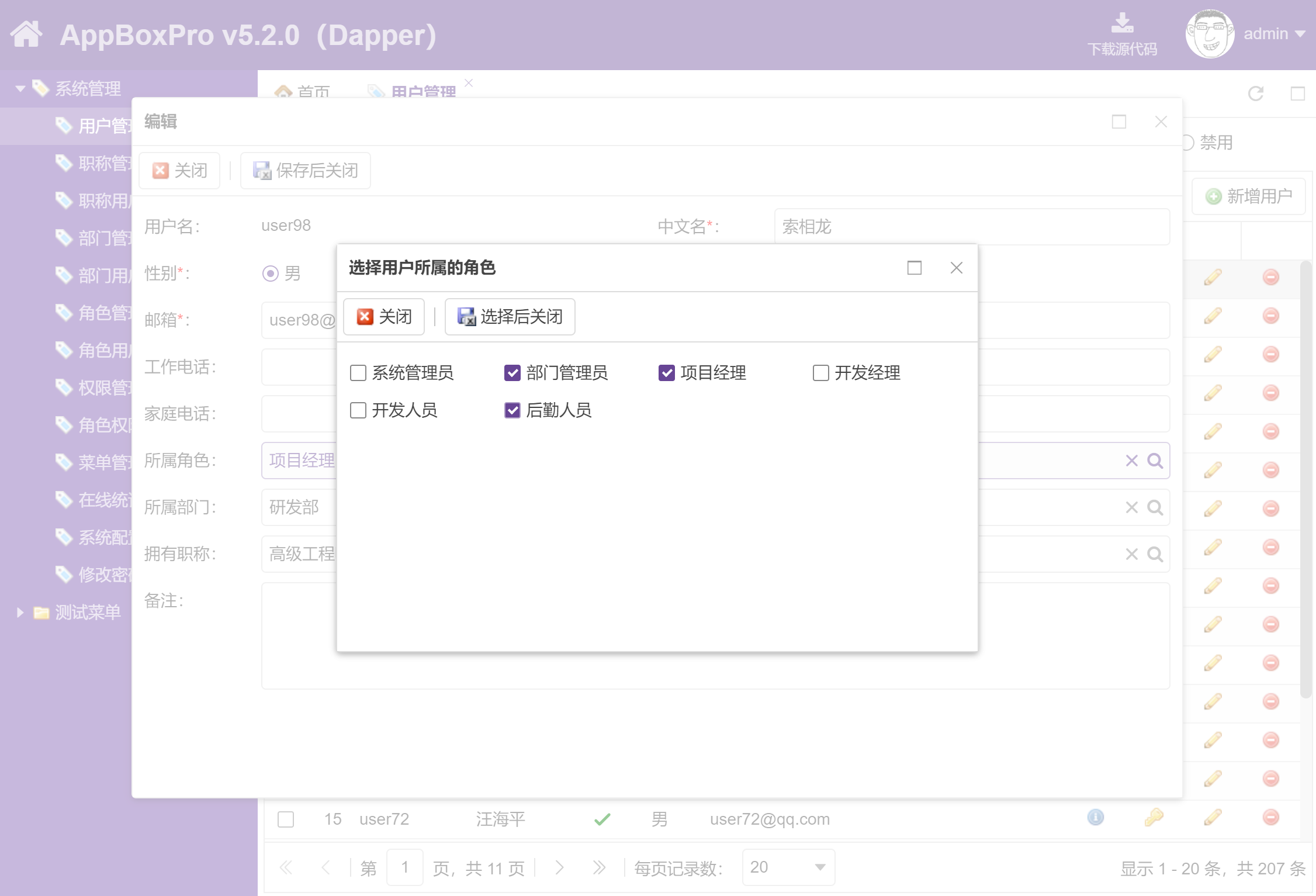# 前言

AppBox的详细介绍：https://www.cnblogs.com/sanshi/p/4030265.html

5 年来，我们一直在津津乐道 Entity Framework 带来的好处，也许是情人眼里出西施，对于它的缺点文过饰非，大可用一句话搪塞：你要完整学习 Entity Framework 知识体系，方能事半功倍，俗话说：磨刀不误砍柴工。

1. 数据库在哪？怎么没有数据库初始脚本？

2. 怎么又出错了？到底执行的SQL语句是啥？

3. 怎么支持 MySQL 数据库？为什么SQLServer正常的查询，到MySQL就出错了？

4. 为啥突然数据库都清空了？好恐怖，幸好不是在服务器

5. 性能怎么样？大家都说EF的性能不好

6. 能不能先建数据库，然后生成模型类？

.....

# 为什么要转到Dapper？

Entity Framework是一个有益的尝试，尝试向开发人员隐藏 SQL 语句，所有的数据库查询操作都通过面向对象的 C# 语言来完成，可以想象，从关系型数据库抽象为面向对象的语言，这个扭曲力场不可谓不强大，而这个扭曲力会带来两个极端：

1. 简单的操作会更加简单

2. 复杂的操作会更加复杂

## 哪些是简单的操作呢？

Entity Framework CodeFirst开发模式允许我们只写模型类，程序会在第一次运行时创建数据库，比如一个简单的用户角色关系，通过模型类可以这么定义：

public class Role : IKeyID
{
[Key]
public int ID { get; set; }

[Required, StringLength(50)]
public string Name { get; set; }

[StringLength(500)]
public string Remark { get; set; }

public virtual ICollection<User> Users { get; set; }

}
public class User : IKeyID
{
[Key]
public int ID { get; set; }

[Required, StringLength(50)]
public string Name { get; set; }

[Required, StringLength(100)]
public string Email { get; set; }

[Required, StringLength(50)]
public string Password { get; set; }

public virtual ICollection<Role> Roles { get; set; }

}

modelBuilder.Entity<Role>()
.HasMany(r => r.Users)
.WithMany(u => u.Roles)
.Map(x => x.ToTable("RoleUsers")
.MapLeftKey("RoleID")
.MapRightKey("UserID"));

1. 一个角色可以有多个用户（HasMany）

2. 一个用户可以有多个角色（WithMany）

3. 将这种关联关系保存到数据库表 RoleUsers，对于两个外键：RoleID和UserID

CREATE TABLE IF NOT EXISTS roles (
ID int(11) NOT NULL AUTO_INCREMENT,
Name varchar(50) CHARACTER  NOT NULL,
Remark varchar(500) CHARACTER  DEFAULT NULL,
PRIMARY KEY (ID),
UNIQUE KEY ID (ID)
);

CREATE TABLE IF NOT EXISTS users (
ID int(11) NOT NULL AUTO_INCREMENT,
Name varchar(50) CHARACTER  NOT NULL,
Email varchar(100) CHARACTER  NOT NULL,
Password varchar(50) CHARACTER  NOT NULL,
Enabled tinyint(1) NOT NULL,
PRIMARY KEY (ID),
UNIQUE KEY ID (ID)
);

CREATE TABLE IF NOT EXISTS roleusers (
RoleID int(11) NOT NULL,
UserID int(11) NOT NULL,
PRIMARY KEY (RoleID,UserID),
KEY Role_Users_Target (UserID),
CONSTRAINT Role_Users_Source FOREIGN KEY (RoleID) REFERENCES roles (id) ON DELETE CASCADE,
CONSTRAINT Role_Users_Target FOREIGN KEY (UserID) REFERENCES users (id) ON DELETE CASCADE
);

1. Role_Users_Source：定义外键 RoleID，关联 roles 表的 ID 列，并使用 ON DELETE CASCADE 定义级联删除，如果roles 表删除了一行数据，那么roleusers 中一行或多行关联数据会被删除

2. Role_Users_Target：定义外键 UserID，关联 users 表的 ID 列，同样定义级联删除规则

DB.Roles.Find(id)

Role item = DB.Roles.Find(id);
item.Name = tbxName.Text.Trim();
item.Remark = tbxRemark.Text.Trim();
DB.SaveChanges();

DB.Roles.Where(r => r.ID == roleID).Delete();

DB.Users.Where(u => u.Roles.Any(r => r.ID == roleID)).Count();

SELECT
[GroupBy1].[A1] AS [C1]
FROM ( SELECT
COUNT(1) AS [A1]
FROM [dbo].[Users] AS [Extent1]
WHERE  EXISTS (SELECT
1 AS [C1]
FROM [dbo].[RoleUsers] AS [Extent2]
WHERE ([Extent1].[ID] = [Extent2].[UserID]) AND ([Extent2].[RoleID] = @p__linq__0)
)
)  AS [GroupBy1]

SELECT
COUNT(1)
FROM [dbo].[Users]
WHERE  EXISTS (SELECT
1 AS [C1]
FROM [dbo].[RoleUsers]
WHERE ([Users].[ID] = [RoleUsers].[UserID]) AND ([RoleUsers].[RoleID] = @p__linq__0)
)

SELECT
COUNT(*)
FROM [dbo].[RoleUsers]
WHERE ([Users].[ID] = [RoleUsers].[UserID]) AND ([RoleUsers].[RoleID] = @p__linq__0)

conn.QuerySingleOrDefault<Role>("select * from roles where ID = @RoleID", new { RoleID = roleID });

Role item = GetCurrentRole(id);
item.Name = tbxName.Text.Trim();
item.Remark = tbxRemark.Text.Trim();

conn.Execute("update roles set Name = @Name, Remark = @Remark where ID = @ID", item);

conn.Execute("delete from roles where ID = @RoleID", new { RoleID = roleID });

conn.QuerySingle<int>("select count(*) from roleusers where RoleID = @RoleID", new { RoleID = roleID });

## 哪些是复杂的操作呢？

int roleID = GetSelectedDataKeyID(Grid1);
List<int> userIDs = GetSelectedDataKeyIDs(Grid2);

Role role = DB.Roles.Include(r => r.Users)
.Where(r => r.ID == roleID)
.FirstOrDefault();

foreach (int userID in userIDs)
{
User user = role.Users.Where(u => u.ID == userID).FirstOrDefault();
if (user != null)
{
role.Users.Remove(user);
}
}

DB.SaveChanges();

1. 首先获取当前角色，由于后面要操作角色的用户列表，所以使用 Include 语句，这将导致生成SQL查询语句有点复杂：

SELECT
[Project2].[ID] AS [ID],
[Project2].[Name] AS [Name],
[Project2].[Remark] AS [Remark],
[Project2].[C1] AS [C1],
[Project2].[ID1] AS [ID1],
[Project2].[Name1] AS [Name1],
FROM ( SELECT
[Limit1].[ID] AS [ID],
[Limit1].[Name] AS [Name],
[Limit1].[Remark] AS [Remark],
[Join1].[ID] AS [ID1],
[Join1].[Name] AS [Name1],
CASE WHEN ([Join1].[RoleID] IS NULL) THEN CAST(NULL AS int) ELSE 1 END AS [C1]
FROM   (SELECT TOP (1)
[Extent1].[ID] AS [ID],
[Extent1].[Name] AS [Name],
[Extent1].[Remark] AS [Remark]
FROM [dbo].[Roles] AS [Extent1]
WHERE [Extent1].[ID] = @p__linq__0 ) AS [Limit1]
LEFT OUTER JOIN  (SELECT [Extent2].[RoleID] AS [RoleID], [Extent3].[ID] AS [ID], [Extent3].[Name] AS [Name]
FROM  [dbo].[RoleUsers] AS [Extent2]
INNER JOIN [dbo].[Users] AS [Extent3] ON [Extent3].[ID] = [Extent2].[UserID] ) AS [Join1] ON [Limit1].[ID] = [Join1].[RoleID]
)  AS [Project2]
ORDER BY [Project2].[ID] ASC, [Project2].[C1] ASC

2. 遍历需要删除的用户列表，并从当前角色的用户列表中删除，这将执行多个SQL语句：

exec sp_executesql N'DELETE [dbo].[RoleUsers]
WHERE (([RoleID] = @0) AND ([UserID] = @1))',N'@0 int,@1 int',@0=3,@1=45
go
exec sp_executesql N'DELETE [dbo].[RoleUsers]
WHERE (([RoleID] = @0) AND ([UserID] = @1))',N'@0 int,@1 int',@0=3,@1=46
go
exec sp_executesql N'DELETE [dbo].[RoleUsers]
WHERE (([RoleID] = @0) AND ([UserID] = @1))',N'@0 int,@1 int',@0=3,@1=47
go

。。。。。

int roleID = GetSelectedDataKeyID(Grid1);
List<int> userIDs = GetSelectedDataKeyIDs(Grid2);

conn.Execute("delete from roleusers where RoleID = @RoleID and UserID in @UserIDs", new { RoleID = roleID, UserIDs = userIDs });

User item = DB.Users
.Include(u => u.Roles)
.Where(u => u.ID == id).FirstOrDefault();

int[] roleIDs = StringUtil.GetIntArrayFromString(hfSelectedRole.Text);
ReplaceEntities<Role>(item.Roles, roleIDs);

DB.SaveChanges();

protected void ReplaceEntities<T>(ICollection<T> existEntities, int[] newEntityIDs) where T : class,  IKeyID, new()
{
if (newEntityIDs.Length == 0)
{
existEntities.Clear();
}
else
{
int[] tobeAdded = newEntityIDs.Except(existEntities.Select(x => x.ID)).ToArray();
int[] tobeRemoved = existEntities.Select(x => x.ID).Except(newEntityIDs).ToArray();

existEntities.Where(x => tobeRemoved.Contains(x.ID)).ToList().ForEach(e => existEntities.Remove(e));
}
}

exec sp_executesql N'DELETE [dbo].[RoleUsers]
WHERE (([RoleID] = @0) AND ([UserID] = @1))',N'@0 int,@1 int',@0=3,@1=50
go
exec sp_executesql N'DELETE [dbo].[RoleUsers]
WHERE (([RoleID] = @0) AND ([UserID] = @1))',N'@0 int,@1 int',@0=23,@1=50
go
exec sp_executesql N'DELETE [dbo].[RoleUsers]
WHERE (([RoleID] = @0) AND ([UserID] = @1))',N'@0 int,@1 int',@0=33,@1=50
go
exec sp_executesql N'INSERT [dbo].[RoleUsers]([RoleID], [UserID])
VALUES (@0, @1)
',N'@0 int,@1 int',@0=4,@1=50
go
exec sp_executesql N'INSERT [dbo].[RoleUsers]([RoleID], [UserID])
VALUES (@0, @1)
',N'@0 int,@1 int',@0=6,@1=50
go
exec sp_executesql N'INSERT [dbo].[RoleUsers]([RoleID], [UserID])
VALUES (@0, @1)
',N'@0 int,@1 int',@0=7,@1=50
go

。。。。。。

User item = DB.Users
.Include(u => u.Roles)
.Where(u => u.ID == id).FirstOrDefault();

int[] roleIDs = StringUtil.GetIntArrayFromString(hfSelectedRole.Text);

conn.Execute("delete from roleusers where UserID = @UserID", new { UserID = userID });
conn.Execute("insert roleusers (UserID, RoleID) values (@UserID, @RoleID)", roleIDs.Select(u => new { UserID = userID, RoleID = u }).ToList());

# 后记

1. 文中提到的 AppBox 不是免费软件，如果需要了解更多详情，请加入【三石和他的朋友们】知识星球下载源代码：http://fineui.com/fans/

2. 取决于本篇博文的受欢迎程度，我可能会写一个续篇，包含更多的升级细节和Dapper的使用技巧：

• 批量更新数据
• 分页与排序的简单封装
• 插入与更新的简单封装
• 事务（Transaction）
• 插入后返回自增ID
• 动态创建匿名参数
• 子查询
• 多结果映射# 【续】5年后，我们为什么要从 Entity Framework 转到 Dapper 工具？

posted @ 2018-08-27 10:50  三生石上(FineUI控件)  阅读(16623)  评论(150编辑  收藏  举报